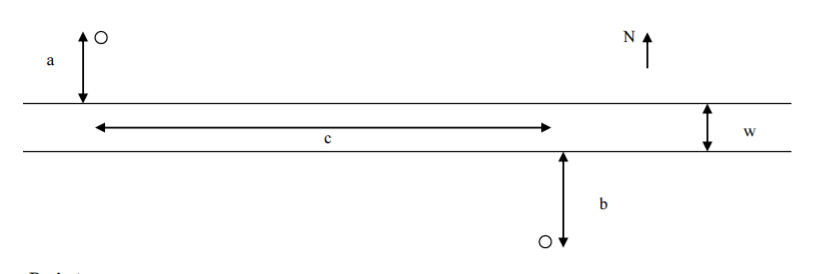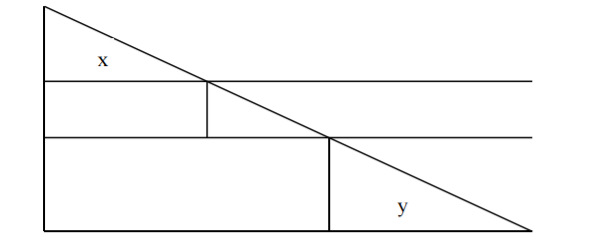# Geometry/Algebra: Model BuildingMy professor gave us a list of problems related to a project.

For the triangle below we have the bigger triangle have dimensions c for width and a + w + b. That would mean that a and b are related to x and y directly and the smallest triangle (middle) has dimensions c - (x+y) and w.

We are supposed to show that connecting the two locations using a single straight line-segment is given by:

$$C_1 = (c_L(a + b) + c_R w) \sqrt{1 + (\frac{c}{a + w + b})^2}$$

Variables: $c_L$ = cost on land $c_R$ = cost on river

the first tip suggest to find x,y and it mentions to use the fact that they share a relationship with the biggest triangle, and to use the Pythagorean theorem to determine the relevant lengths. It suggest that I find what

$\frac{x}{a}$ and $\frac{y}{b}$ are equal too. I believe that both should be equal to:

$$\frac{x}{a} = \frac{y}{b} = \frac{c}{a + w + b}$$

It then states after factoring out $a, b, w$ from the square root, the cost $c_1$ of connecting the two locations using a single straight line-segment is given by the following which I am supposed to prove.

$$C_1 = (c_L(\quad) + c_R w) \sqrt{1 + \quad}$$

So I am not sure how I am supposed to approach this. I am not even sure why the relationship $\frac{x}{a}$ and $\frac{y}{b}$ is important.

• The entire project is based off of this first questions so any help would be greatly appreciated – Hawaiian Rolls Feb 5 '17 at 23:20

## 1 Answer

This question is beautiful.Now some things should be obvious from this picture namely that your cost function is

$$C= C_L\Big[\sqrt{a^2+x^2}+\sqrt{b^2+y^2}\Big]+C_R\Big[\sqrt{w^2+(c-x-y)^2}\Big]$$

Which can be rewriten as

$$C_L\Big[a\sqrt{1+(\frac{x}{a})^2}+b\sqrt{1+(\frac{y}{b})^2}\Big]+C_R\Big[w\sqrt{1+(\frac{c-x-y}{w})^2}\Big]$$

Another thing to notice is that

$$\frac{x}{a}=\frac{c-x-y}{w}=\frac{y}{b}=\frac{c}{a+w+b}$$

• Thank you so much. I thought that I had to pull $a, w, b$ from the same square root not different roots. Although the inputs for a and b are not in the possible answer sheet that our professor provided he did say we would have to come up with some on our own and your logic and everything looks pretty good here. I want to thank you as I have been stuck on this for the past 6 hours thinking that it was this complex problem. Pythagorean and those triangles! – Hawaiian Rolls Feb 6 '17 at 3:40
• If you want to see the rest of the project let me know! I'm in a model building class and don't mind sharing the fun exercises! – Hawaiian Rolls Feb 6 '17 at 3:42
• @HawaiianRolls Sure. – user160110 Feb 6 '17 at 3:42
• It's the beginning weeks of class, so this is the first project that we have. I am only on the first problem, but you have fun! dropbox.com/sh/qfn5lc3ctft6d4k/AABoiZLd7WYjuT8RRRZWZSDna?dl=0 – Hawaiian Rolls Feb 6 '17 at 3:45